Next: Stationary States Up: Wave Mechanics Previous: Heisenberg's Uncertainty Principle

# Wavefunction Collapse

Consider a spatially extended wavefunction,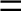. According to our usual interpretation,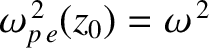is proportional to the probability of a measurement of the particle's position yielding a value in the range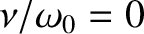to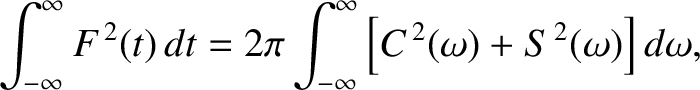at time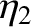. Thus, if the wavefunction is extended then there is a wide range of likely values that such a measurement could give. Suppose, however, that we make a measurement of the particle's position, and obtain the value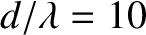. We now know that the particle is located at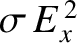. If we make another measurement, immediately after the first one, then what value would we expect to obtain? Common sense tells us that we should obtain the same value,, because the particle cannot have shifted position appreciably in an infinitesimal time interval. Thus, immediately after the first measurement, a measurement of the particle's position is certain to give the value, and has no chance of giving any other value. This implies that the wavefunction must have collapsed to some sort of spike'' function, centered on. This idea is illustrated in Figure 80. As soon as the wavefunction collapses, it starts to expand again, as described in the previous section. Thus, the second measurement must be made reasonably quickly after the first one, otherwise the same result will not necessarily be obtained.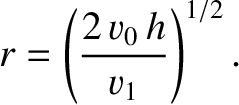The preceding discussion illustrates an important point in wave mechanics. That is, the wavefunction of a massive particle changes discontinuously (in time) whenever a measurement of the particle's position is made. We conclude that there are two types of time evolution of the wavefunction in wave mechanics. First, there is a smooth evolution that is governed by Schrödinger's equation. This evolution takes place between measurements. Second, there is a discontinuous evolution that takes place each time a measurement is made.Next: Stationary States Up: Wave Mechanics Previous: Heisenberg's Uncertainty Principle
Richard Fitzpatrick 2013-04-08